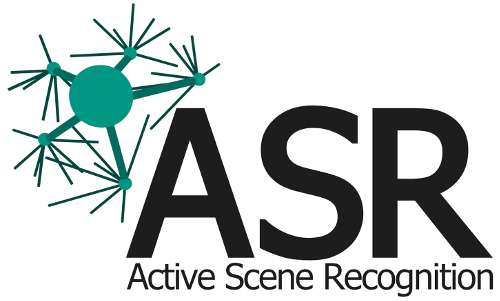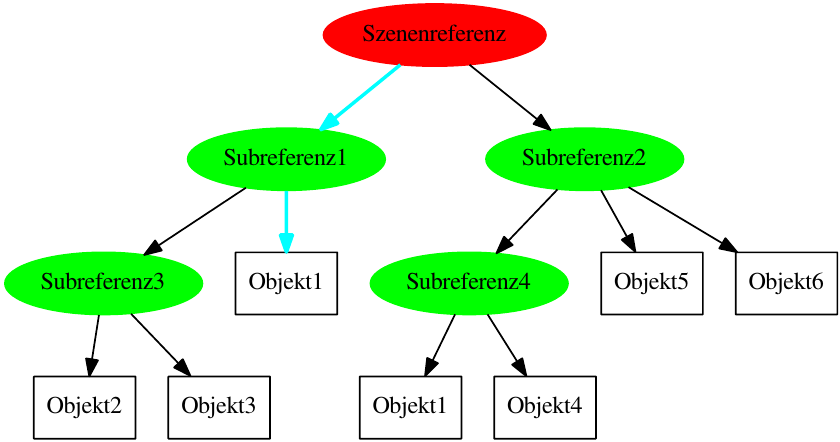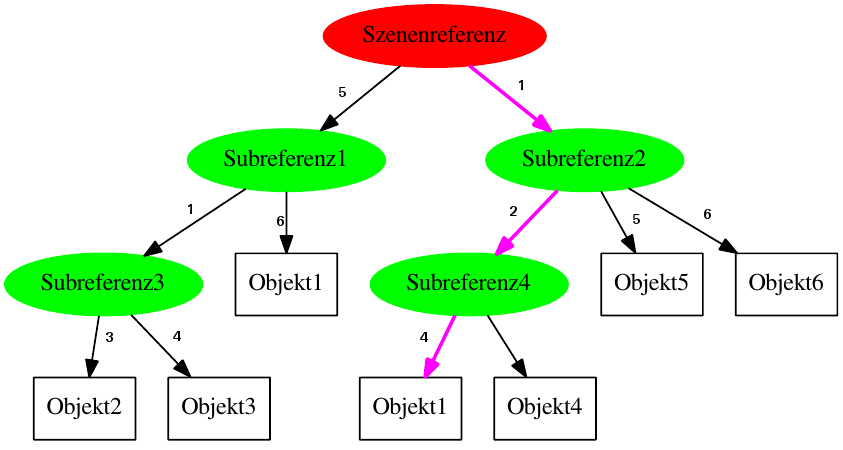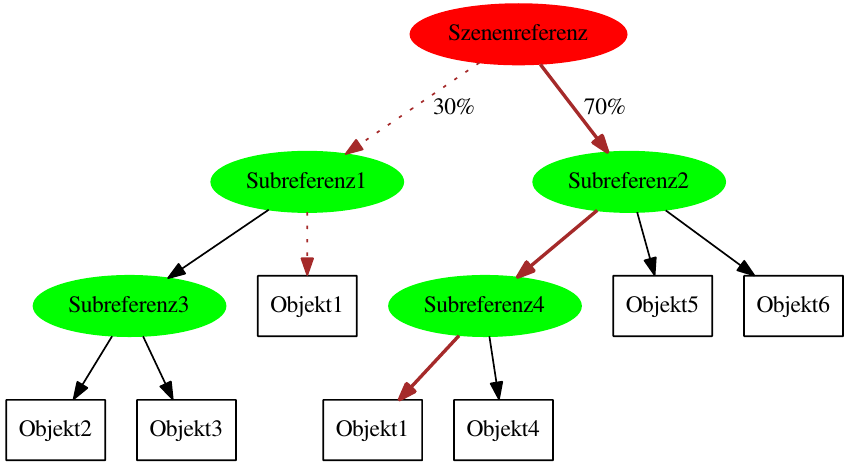[Documentation] [TitleIndex] [WordIndex]

# Package Summary

This package contains classes and algorithms to predict poses of searched objects by the help of a tree of ISMs. It is organized as a library and contains only a small program to evaluate the performance of different algorithms.

# Package Summary

This package contains classes and algorithms to predict poses of searched objects by the help of a tree of ISMs. It is organized as a library and contains only a small program to evaluate the performance of different algorithms.# Description

This package contains classes and algorithms to predict poses of searched objects by the help of a tree of ISMs. It is organized as a library and contains only a small program to evaluate the performance of different algorithms.

# Functionality

The main functionality of this package is prediction missing or unfound objects in an ISM-scene. It provides four algorithms for solving the problem:

• shortest path: This algorithm uses Breadth-first search to find a paths to all unfound objects. Which path to choose depends on the length of the path. The following picture illustrates this. In example, "Object 1" can be found in "Subrefernce 1" and "Subreference 4". The algorithm will return all predicted poses of "Object 1" from "Subreference 1" because the path to it is shorter.• best path: This algorithm uses Breadth-first search and a goodness function to find a paths to all unfound objects. Which path to choose depends on the goodness. Therefore, each path gets costs assigned. The algorithm chooses the path with the best goodness (=least costs). This is shown in the following pictures. The numbers on the paths are the costs. The algorithm will return all predicted poses of "Subreference 4", because the path is better (Cost for "Subreference 1"=11; Cost for "Subreference 4"=7):• random path: This algorithm evaluates all paths from reference to a missing object and picks randomly one due prediciton. The probability for a path depends on the path's goodness (The higher the goodness, the higher the probability). The following picture shows this. Like in the example above, the path to "Subreference 4" is better. Due to that, it has a higher probability. The algorithm will return 30% of the predicted poses form "Subreference 1" and 70 % from "Subreference 4":• old (non) normalized: old naive pose prediction. It is not recommended to use it due its bad performance.

It is very important to set the parameter "sampleFactor" wisely (see Section Tutorial). The sampleFactor indirectly affects the returned number of object poses. This is calculated with numberOfTrainingSetsInDB * sampleFactor = numberOfObjectPoses. E.g. your DB contains 200 datasets, and your sampleFactor = 0.5, the algorithm will predict 100 object poses.

# Usage

To use the lib simply included the necessary header files, choose the desired algorithm and see the code tutorial described below.

## Needed packages

• asr_lib_ism: for complex ISM-data-structure and data-base connection

• asr_msgs: basic data-structure and messages

## Start system

It is a libery, so you cant run it on itself. But you can run the test-node by calling. Thereforce you have included the executable in your !CMakeLists.txt and recompiled the package. Make sure, that all parameters (data-base folder, output-path and sample-rate) are set in the launch-file aforementioned.

# Tutorials

#include <asr_msgs/AsrObject.h>

#include <pose_prediction_ism/pose_predictor.h> #include <pose_prediction_ism/shortest_path.h>

pose_prediction_ism::PosePredictor* pose_predictor;

//path to database std::string db_filename = PATH_TO_YOUR_DATABASE;

//use shortest path pose prediction. You can also use BestPath, RandomPath, PaperPredictionNormalized or PaperPredictionNonNormalized pose_predictor = new pose_prediction_ism::ShortestPath(db_filename);

//enables the random functionallity to simulate some noise (in mm, deg) //pose_predictor_->enableRandom(random_position, random_orientation);

//Buffer for already found objects in scene pose_prediction_ism::FoundObjects fos;

//add all already found objects to buffer for(std::size_t i = 0; i < BUFFERED_OBJECTS.size(); i++) { asr_msgs::AsrObject asr_o; asr_o.type = BUFFERED_OBJECTS[i]->type; asr_o.identifier = BUFFERED_OBJECTS[i]->observedId; fos.push_back(asr_o); }

//ISM::PosePtr of ISM reference ISM::PosePtr referencePosePtr = MY_REFERENCE;

//name of pattern representing ISM std::string = "MyPattern";

//factor of votes for number of predicted poses double samplefactor = 1.0;

//call actually prediction here with parameters: pose_predictor_->predictUnfoundPoses(referencePosePtr, pattern_name, samplefactor);

//get the resulting attributed point cloud (which contains the prediction-results as labed points) pose_prediction_ism::AttributedPointCloud current_point_cloud = pose_predictor_->getAttributedPointCloud();

//disables the random functionallity //pose_predictor_->disableRandom();

2021-01-23 12:32